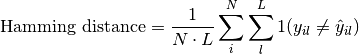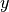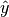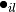Shortcuts

# Hamming Distance¶

## Module Interface¶

### HammingDistance¶

class torchmetrics.HammingDistance(task: Literal['binary', 'multiclass', 'multilabel'], threshold: float = 0.5, num_classes: = None, num_labels: = None, average: Optional[Literal['micro', 'macro', 'weighted', 'none']] = 'micro', multidim_average: Optional[Literal['global', 'samplewise']] = 'global', top_k: = 1, ignore_index: = None, validate_args: bool = True, **kwargs: Any)[source]

Computes the average Hamming distance (also known as Hamming loss):Whereis a tensor of target values,is a tensor of predictions, andrefers to the-th label of the-th sample of that tensor.

This function is a simple wrapper to get the task specific versions of this metric, which is done by setting the task argument to either 'binary', 'multiclass' or multilabel. See the documentation of BinaryHammingDistance, MulticlassHammingDistance and MultilabelHammingDistance for the specific details of each argument influence and examples.

Legacy Example:
>>> target = torch.tensor([[0, 1], [1, 1]])
>>> preds = torch.tensor([[0, 1], [0, 1]])
>>> hamming_distance(preds, target)
tensor(0.2500)


### BinaryHammingDistance¶

class torchmetrics.classification.BinaryHammingDistance(threshold=0.5, multidim_average='global', ignore_index=None, validate_args=True, **kwargs)[source]

Computes the average Hamming distance (also known as Hamming loss) for binary tasks:Whereis a tensor of target values,is a tensor of predictions, andrefers to the-th label of the-th sample of that tensor.

As input to forward and update the metric accepts the following input:

• preds (Tensor): An int or float tensor of shape (N, ...). If preds is a floating point tensor with values outside [0,1] range we consider the input to be logits and will auto apply sigmoid per element. Addtionally, we convert to int tensor with thresholding using the value in threshold.

• target (Tensor): An int tensor of shape (N, ...).

As output to forward and compute the metric returns the following output:

• bhd (Tensor): A tensor whose returned shape depends on the multidim_average arguments:

• If multidim_average is set to global, the metric returns a scalar value.

• If multidim_average is set to samplewise, the metric returns (N,) vector consisting of a scalar value per sample.

Parameters
• threshold (float) – Threshold for transforming probability to binary {0,1} predictions

• multidim_average (Literal[‘global’, ‘samplewise’]) –

Defines how additionally dimensions ... should be handled. Should be one of the following:

• global: Additional dimensions are flatted along the batch dimension

• samplewise: Statistic will be calculated independently for each sample on the N axis. The statistics in this case are calculated over the additional dimensions.

• ignore_index (Optional[int]) – Specifies a target value that is ignored and does not contribute to the metric calculation

• validate_args (bool) – bool indicating if input arguments and tensors should be validated for correctness. Set to False for faster computations.

Example (preds is int tensor):
>>> from torchmetrics.classification import BinaryHammingDistance
>>> target = torch.tensor([0, 1, 0, 1, 0, 1])
>>> preds = torch.tensor([0, 0, 1, 1, 0, 1])
>>> metric = BinaryHammingDistance()
>>> metric(preds, target)
tensor(0.3333)

Example (preds is float tensor):
>>> from torchmetrics.classification import BinaryHammingDistance
>>> target = torch.tensor([0, 1, 0, 1, 0, 1])
>>> preds = torch.tensor([0.11, 0.22, 0.84, 0.73, 0.33, 0.92])
>>> metric = BinaryHammingDistance()
>>> metric(preds, target)
tensor(0.3333)

Example (multidim tensors):
>>> from torchmetrics.classification import BinaryHammingDistance
>>> target = torch.tensor([[[0, 1], [1, 0], [0, 1]], [[1, 1], [0, 0], [1, 0]]])
>>> preds = torch.tensor(
...     [
...         [[0.59, 0.91], [0.91, 0.99], [0.63, 0.04]],
...         [[0.38, 0.04], [0.86, 0.780], [0.45, 0.37]],
...     ]
... )
>>> metric = BinaryHammingDistance(multidim_average='samplewise')
>>> metric(preds, target)
tensor([0.6667, 0.8333])


Initializes internal Module state, shared by both nn.Module and ScriptModule.

### MulticlassHammingDistance¶

class torchmetrics.classification.MulticlassHammingDistance(num_classes, top_k=1, average='macro', multidim_average='global', ignore_index=None, validate_args=True, **kwargs)[source]

Computes the average Hamming distance (also known as Hamming loss) for multiclass tasks:Whereis a tensor of target values,is a tensor of predictions, andrefers to the-th label of the-th sample of that tensor.

As input to forward and update the metric accepts the following input:

• preds (Tensor): An int tensor of shape (N, ...) or float tensor of shape (N, C, ..). If preds is a floating point we apply torch.argmax along the C dimension to automatically convert probabilities/logits into an int tensor.

• target (Tensor): An int tensor of shape (N, ...).

As output to forward and compute the metric returns the following output:

• mchd (Tensor): A tensor whose returned shape depends on the average and multidim_average arguments:

• If multidim_average is set to global:

• If average='micro'/'macro'/'weighted', the output will be a scalar tensor

• If average=None/'none', the shape will be (C,)

• If multidim_average is set to samplewise:

• If average='micro'/'macro'/'weighted', the shape will be (N,)

• If average=None/'none', the shape will be (N, C)

Parameters
• num_classes (int) – Integer specifing the number of classes

• average (Optional[Literal[‘micro’, ‘macro’, ‘weighted’, ‘none’]]) –

Defines the reduction that is applied over labels. Should be one of the following:

• micro: Sum statistics over all labels

• macro: Calculate statistics for each label and average them

• weighted: Calculates statistics for each label and computes weighted average using their support

• "none" or None: Calculates statistic for each label and applies no reduction

• top_k (int) – Number of highest probability or logit score predictions considered to find the correct label. Only works when preds contain probabilities/logits.

• multidim_average (Literal[‘global’, ‘samplewise’]) –

Defines how additionally dimensions ... should be handled. Should be one of the following:

• global: Additional dimensions are flatted along the batch dimension

• samplewise: Statistic will be calculated independently for each sample on the N axis. The statistics in this case are calculated over the additional dimensions.

• ignore_index (Optional[int]) – Specifies a target value that is ignored and does not contribute to the metric calculation

• validate_args (bool) – bool indicating if input arguments and tensors should be validated for correctness. Set to False for faster computations.

Example (preds is int tensor):
>>> from torchmetrics.classification import MulticlassHammingDistance
>>> target = torch.tensor([2, 1, 0, 0])
>>> preds = torch.tensor([2, 1, 0, 1])
>>> metric = MulticlassHammingDistance(num_classes=3)
>>> metric(preds, target)
tensor(0.1667)
>>> mchd = MulticlassHammingDistance(num_classes=3, average=None)
>>> mchd(preds, target)
tensor([0.5000, 0.0000, 0.0000])

Example (preds is float tensor):
>>> from torchmetrics.classification import MulticlassHammingDistance
>>> target = torch.tensor([2, 1, 0, 0])
>>> preds = torch.tensor([
...   [0.16, 0.26, 0.58],
...   [0.22, 0.61, 0.17],
...   [0.71, 0.09, 0.20],
...   [0.05, 0.82, 0.13],
... ])
>>> metric = MulticlassHammingDistance(num_classes=3)
>>> metric(preds, target)
tensor(0.1667)
>>> mchd = MulticlassHammingDistance(num_classes=3, average=None)
>>> mchd(preds, target)
tensor([0.5000, 0.0000, 0.0000])

Example (multidim tensors):
>>> from torchmetrics.classification import MulticlassHammingDistance
>>> target = torch.tensor([[[0, 1], [2, 1], [0, 2]], [[1, 1], [2, 0], [1, 2]]])
>>> preds = torch.tensor([[[0, 2], [2, 0], [0, 1]], [[2, 2], [2, 1], [1, 0]]])
>>> metric = MulticlassHammingDistance(num_classes=3, multidim_average='samplewise')
>>> metric(preds, target)
tensor([0.5000, 0.7222])
>>> mchd = MulticlassHammingDistance(num_classes=3, multidim_average='samplewise', average=None)
>>> mchd(preds, target)
tensor([[0.0000, 1.0000, 0.5000],
[1.0000, 0.6667, 0.5000]])


Initializes internal Module state, shared by both nn.Module and ScriptModule.

### MultilabelHammingDistance¶

class torchmetrics.classification.MultilabelHammingDistance(num_labels, threshold=0.5, average='macro', multidim_average='global', ignore_index=None, validate_args=True, **kwargs)[source]

Computes the average Hamming distance (also known as Hamming loss) for multilabel tasks:Whereis a tensor of target values,is a tensor of predictions, andrefers to the-th label of the-th sample of that tensor.

As input to forward and update the metric accepts the following input:

• preds (Tensor): An int tensor or float tensor of shape (N, C, ...). If preds is a floating point tensor with values outside [0,1] range we consider the input to be logits and will auto apply sigmoid per element. Addtionally, we convert to int tensor with thresholding using the value in threshold.

• target (Tensor): An int tensor of shape (N, C, ...).

As output to forward and compute the metric returns the following output:

• mlhd (Tensor): A tensor whose returned shape depends on the average and multidim_average arguments:

• If multidim_average is set to global:

• If average='micro'/'macro'/'weighted', the output will be a scalar tensor

• If average=None/'none', the shape will be (C,)

• If multidim_average is set to samplewise:

• If average='micro'/'macro'/'weighted', the shape will be (N,)

• If average=None/'none', the shape will be (N, C)

Parameters
• num_labels (int) – Integer specifing the number of labels

• threshold (float) – Threshold for transforming probability to binary (0,1) predictions

• average (Optional[Literal[‘micro’, ‘macro’, ‘weighted’, ‘none’]]) –

Defines the reduction that is applied over labels. Should be one of the following:

• micro: Sum statistics over all labels

• macro: Calculate statistics for each label and average them

• weighted: Calculates statistics for each label and computes weighted average using their support

• "none" or None: Calculates statistic for each label and applies no reduction

• multidim_average (Literal[‘global’, ‘samplewise’]) –

Defines how additionally dimensions ... should be handled. Should be one of the following:

• global: Additional dimensions are flatted along the batch dimension

• samplewise: Statistic will be calculated independently for each sample on the N axis. The statistics in this case are calculated over the additional dimensions.

• ignore_index (Optional[int]) – Specifies a target value that is ignored and does not contribute to the metric calculation

• validate_args (bool) – bool indicating if input arguments and tensors should be validated for correctness. Set to False for faster computations.

Example (preds is int tensor):
>>> from torchmetrics.classification import MultilabelHammingDistance
>>> target = torch.tensor([[0, 1, 0], [1, 0, 1]])
>>> preds = torch.tensor([[0, 0, 1], [1, 0, 1]])
>>> metric = MultilabelHammingDistance(num_labels=3)
>>> metric(preds, target)
tensor(0.3333)
>>> mlhd = MultilabelHammingDistance(num_labels=3, average=None)
>>> mlhd(preds, target)
tensor([0.0000, 0.5000, 0.5000])

Example (preds is float tensor):
>>> from torchmetrics.classification import MultilabelHammingDistance
>>> target = torch.tensor([[0, 1, 0], [1, 0, 1]])
>>> preds = torch.tensor([[0.11, 0.22, 0.84], [0.73, 0.33, 0.92]])
>>> metric = MultilabelHammingDistance(num_labels=3)
>>> metric(preds, target)
tensor(0.3333)
>>> mlhd = MultilabelHammingDistance(num_labels=3, average=None)
>>> mlhd(preds, target)
tensor([0.0000, 0.5000, 0.5000])

Example (multidim tensors):
>>> from torchmetrics.classification import MultilabelHammingDistance
>>> target = torch.tensor([[[0, 1], [1, 0], [0, 1]], [[1, 1], [0, 0], [1, 0]]])
>>> preds = torch.tensor(
...     [
...         [[0.59, 0.91], [0.91, 0.99], [0.63, 0.04]],
...         [[0.38, 0.04], [0.86, 0.780], [0.45, 0.37]],
...     ]
... )
>>> metric = MultilabelHammingDistance(num_labels=3, multidim_average='samplewise')
>>> metric(preds, target)
tensor([0.6667, 0.8333])
>>> mlhd = MultilabelHammingDistance(num_labels=3, multidim_average='samplewise', average=None)
>>> mlhd(preds, target)
tensor([[0.5000, 0.5000, 1.0000],
[1.0000, 1.0000, 0.5000]])


Initializes internal Module state, shared by both nn.Module and ScriptModule.

## Functional Interface¶

### hamming_distance¶

torchmetrics.functional.hamming_distance(preds, target, task, threshold=0.5, num_classes=None, num_labels=None, average='micro', multidim_average='global', top_k=1, ignore_index=None, validate_args=True)[source]

Computes the average Hamming distance (also known as Hamming loss):Whereis a tensor of target values,is a tensor of predictions, andrefers to the-th label of the-th sample of that tensor.

This function is a simple wrapper to get the task specific versions of this metric, which is done by setting the task argument to either 'binary', 'multiclass' or multilabel. See the documentation of binary_hamming_distance(), multiclass_hamming_distance() and multilabel_hamming_distance() for the specific details of each argument influence and examples.

Legacy Example:
>>> target = torch.tensor([[0, 1], [1, 1]])
>>> preds = torch.tensor([[0, 1], [0, 1]])
tensor(0.2500)

Return type

Tensor

### binary_hamming_distance¶

torchmetrics.functional.classification.binary_hamming_distance(preds, target, threshold=0.5, multidim_average='global', ignore_index=None, validate_args=True)[source]

Computes the average Hamming distance (also known as Hamming loss) for binary tasks:Whereis a tensor of target values,is a tensor of predictions, andrefers to the-th label of the-th sample of that tensor.

Accepts the following input tensors:

• preds (int or float tensor): (N, ...). If preds is a floating point tensor with values outside [0,1] range we consider the input to be logits and will auto apply sigmoid per element. Addtionally, we convert to int tensor with thresholding using the value in threshold.

• target (int tensor): (N, ...)

Parameters
• preds (Tensor) – Tensor with predictions

• target (Tensor) – Tensor with true labels

• threshold (float) – Threshold for transforming probability to binary {0,1} predictions

• multidim_average (Literal[‘global’, ‘samplewise’]) –

Defines how additionally dimensions ... should be handled. Should be one of the following:

• global: Additional dimensions are flatted along the batch dimension

• samplewise: Statistic will be calculated independently for each sample on the N axis. The statistics in this case are calculated over the additional dimensions.

• ignore_index (Optional[int]) – Specifies a target value that is ignored and does not contribute to the metric calculation

• validate_args (bool) – bool indicating if input arguments and tensors should be validated for correctness. Set to False for faster computations.

Return type

Tensor

Returns

If multidim_average is set to global, the metric returns a scalar value. If multidim_average is set to samplewise, the metric returns (N,) vector consisting of a scalar value per sample.

Example (preds is int tensor):
>>> from torchmetrics.functional.classification import binary_hamming_distance
>>> target = torch.tensor([0, 1, 0, 1, 0, 1])
>>> preds = torch.tensor([0, 0, 1, 1, 0, 1])
>>> binary_hamming_distance(preds, target)
tensor(0.3333)

Example (preds is float tensor):
>>> from torchmetrics.functional.classification import binary_hamming_distance
>>> target = torch.tensor([0, 1, 0, 1, 0, 1])
>>> preds = torch.tensor([0.11, 0.22, 0.84, 0.73, 0.33, 0.92])
>>> binary_hamming_distance(preds, target)
tensor(0.3333)

Example (multidim tensors):
>>> from torchmetrics.functional.classification import binary_hamming_distance
>>> target = torch.tensor([[[0, 1], [1, 0], [0, 1]], [[1, 1], [0, 0], [1, 0]]])
>>> preds = torch.tensor(
...     [
...         [[0.59, 0.91], [0.91, 0.99], [0.63, 0.04]],
...         [[0.38, 0.04], [0.86, 0.780], [0.45, 0.37]],
...     ]
... )
>>> binary_hamming_distance(preds, target, multidim_average='samplewise')
tensor([0.6667, 0.8333])


### multiclass_hamming_distance¶

torchmetrics.functional.classification.multiclass_hamming_distance(preds, target, num_classes, average='macro', top_k=1, multidim_average='global', ignore_index=None, validate_args=True)[source]

Computes the average Hamming distance (also known as Hamming loss) for multiclass tasks:Whereis a tensor of target values,is a tensor of predictions, andrefers to the-th label of the-th sample of that tensor.

Accepts the following input tensors:

• preds: (N, ...) (int tensor) or (N, C, ..) (float tensor). If preds is a floating point we apply torch.argmax along the C dimension to automatically convert probabilities/logits into an int tensor.

• target (int tensor): (N, ...)

Parameters
• preds (Tensor) – Tensor with predictions

• target (Tensor) – Tensor with true labels

• num_classes (int) – Integer specifing the number of classes

• average (Optional[Literal[‘micro’, ‘macro’, ‘weighted’, ‘none’]]) –

Defines the reduction that is applied over labels. Should be one of the following:

• micro: Sum statistics over all labels

• macro: Calculate statistics for each label and average them

• weighted: Calculates statistics for each label and computes weighted average using their support

• "none" or None: Calculates statistic for each label and applies no reduction

• top_k (int) – Number of highest probability or logit score predictions considered to find the correct label. Only works when preds contain probabilities/logits.

• multidim_average (Literal[‘global’, ‘samplewise’]) –

Defines how additionally dimensions ... should be handled. Should be one of the following:

• global: Additional dimensions are flatted along the batch dimension

• samplewise: Statistic will be calculated independently for each sample on the N axis. The statistics in this case are calculated over the additional dimensions.

• ignore_index (Optional[int]) – Specifies a target value that is ignored and does not contribute to the metric calculation

• validate_args (bool) – bool indicating if input arguments and tensors should be validated for correctness. Set to False for faster computations.

Returns

• If multidim_average is set to global:

• If average='micro'/'macro'/'weighted', the output will be a scalar tensor

• If average=None/'none', the shape will be (C,)

• If multidim_average is set to samplewise:

• If average='micro'/'macro'/'weighted', the shape will be (N,)

• If average=None/'none', the shape will be (N, C)

Return type

The returned shape depends on the average and multidim_average arguments

Example (preds is int tensor):
>>> from torchmetrics.functional.classification import multiclass_hamming_distance
>>> target = torch.tensor([2, 1, 0, 0])
>>> preds = torch.tensor([2, 1, 0, 1])
>>> multiclass_hamming_distance(preds, target, num_classes=3)
tensor(0.1667)
>>> multiclass_hamming_distance(preds, target, num_classes=3, average=None)
tensor([0.5000, 0.0000, 0.0000])

Example (preds is float tensor):
>>> from torchmetrics.functional.classification import multiclass_hamming_distance
>>> target = torch.tensor([2, 1, 0, 0])
>>> preds = torch.tensor([
...   [0.16, 0.26, 0.58],
...   [0.22, 0.61, 0.17],
...   [0.71, 0.09, 0.20],
...   [0.05, 0.82, 0.13],
... ])
>>> multiclass_hamming_distance(preds, target, num_classes=3)
tensor(0.1667)
>>> multiclass_hamming_distance(preds, target, num_classes=3, average=None)
tensor([0.5000, 0.0000, 0.0000])

Example (multidim tensors):
>>> from torchmetrics.functional.classification import multiclass_hamming_distance
>>> target = torch.tensor([[[0, 1], [2, 1], [0, 2]], [[1, 1], [2, 0], [1, 2]]])
>>> preds = torch.tensor([[[0, 2], [2, 0], [0, 1]], [[2, 2], [2, 1], [1, 0]]])
>>> multiclass_hamming_distance(preds, target, num_classes=3, multidim_average='samplewise')
tensor([0.5000, 0.7222])
>>> multiclass_hamming_distance(preds, target, num_classes=3, multidim_average='samplewise', average=None)
tensor([[0.0000, 1.0000, 0.5000],
[1.0000, 0.6667, 0.5000]])


### multilabel_hamming_distance¶

torchmetrics.functional.classification.multilabel_hamming_distance(preds, target, num_labels, threshold=0.5, average='macro', multidim_average='global', ignore_index=None, validate_args=True)[source]

Computes the average Hamming distance (also known as Hamming loss) for multilabel tasks:Whereis a tensor of target values,is a tensor of predictions, andrefers to the-th label of the-th sample of that tensor.

Accepts the following input tensors:

• preds (int or float tensor): (N, C, ...). If preds is a floating point tensor with values outside [0,1] range we consider the input to be logits and will auto apply sigmoid per element. Addtionally, we convert to int tensor with thresholding using the value in threshold.

• target (int tensor): (N, C, ...)

Parameters
• preds (Tensor) – Tensor with predictions

• target (Tensor) – Tensor with true labels

• num_labels (int) – Integer specifing the number of labels

• threshold (float) – Threshold for transforming probability to binary (0,1) predictions

• average (Optional[Literal[‘micro’, ‘macro’, ‘weighted’, ‘none’]]) –

Defines the reduction that is applied over labels. Should be one of the following:

• micro: Sum statistics over all labels

• macro: Calculate statistics for each label and average them

• weighted: Calculates statistics for each label and computes weighted average using their support

• "none" or None: Calculates statistic for each label and applies no reduction

• multidim_average (Literal[‘global’, ‘samplewise’]) –

Defines how additionally dimensions ... should be handled. Should be one of the following:

• global: Additional dimensions are flatted along the batch dimension

• samplewise: Statistic will be calculated independently for each sample on the N axis. The statistics in this case are calculated over the additional dimensions.

• ignore_index (Optional[int]) – Specifies a target value that is ignored and does not contribute to the metric calculation

• validate_args (bool) – bool indicating if input arguments and tensors should be validated for correctness. Set to False for faster computations.

Returns

• If multidim_average is set to global:

• If average='micro'/'macro'/'weighted', the output will be a scalar tensor

• If average=None/'none', the shape will be (C,)

• If multidim_average is set to samplewise:

• If average='micro'/'macro'/'weighted', the shape will be (N,)

• If average=None/'none', the shape will be (N, C)

Return type

The returned shape depends on the average and multidim_average arguments

Example (preds is int tensor):
>>> from torchmetrics.functional.classification import multilabel_hamming_distance
>>> target = torch.tensor([[0, 1, 0], [1, 0, 1]])
>>> preds = torch.tensor([[0, 0, 1], [1, 0, 1]])
>>> multilabel_hamming_distance(preds, target, num_labels=3)
tensor(0.3333)
>>> multilabel_hamming_distance(preds, target, num_labels=3, average=None)
tensor([0.0000, 0.5000, 0.5000])

Example (preds is float tensor):
>>> from torchmetrics.functional.classification import multilabel_hamming_distance
>>> target = torch.tensor([[0, 1, 0], [1, 0, 1]])
>>> preds = torch.tensor([[0.11, 0.22, 0.84], [0.73, 0.33, 0.92]])
>>> multilabel_hamming_distance(preds, target, num_labels=3)
tensor(0.3333)
>>> multilabel_hamming_distance(preds, target, num_labels=3, average=None)
tensor([0.0000, 0.5000, 0.5000])

Example (multidim tensors):
>>> from torchmetrics.functional.classification import multilabel_hamming_distance
>>> target = torch.tensor([[[0, 1], [1, 0], [0, 1]], [[1, 1], [0, 0], [1, 0]]])
>>> preds = torch.tensor(
...     [
...         [[0.59, 0.91], [0.91, 0.99], [0.63, 0.04]],
...         [[0.38, 0.04], [0.86, 0.780], [0.45, 0.37]],
...     ]
... )
>>> multilabel_hamming_distance(preds, target, num_labels=3, multidim_average='samplewise')
tensor([0.6667, 0.8333])
>>> multilabel_hamming_distance(preds, target, num_labels=3, multidim_average='samplewise', average=None)
tensor([[0.5000, 0.5000, 1.0000],
[1.0000, 1.0000, 0.5000]])


© Copyright Copyright (c) 2020-2023, Lightning-AI et al... Revision 825d17f3.

Built with Sphinx using a theme provided by Read the Docs.
Versions
latest
stable
v0.11.3
v0.11.2
v0.11.1
v0.11.0
v0.10.3
v0.10.2
v0.10.1
v0.10.0
v0.9.3
v0.9.2
v0.9.1
v0.9.0
v0.8.2
v0.8.1
v0.8.0
v0.7.3
v0.7.2
v0.7.1
v0.7.0
v0.6.2
v0.6.1
v0.6.0
v0.5.1
v0.5.0
v0.4.1
v0.4.0
v0.3.2
v0.3.1
v0.3.0
v0.2.0
v0.1.0